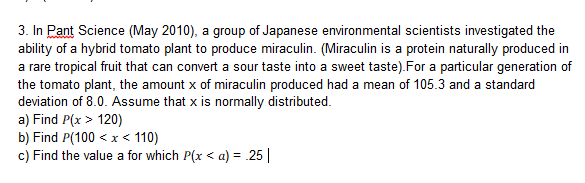3. In Pant Science (May 2010), a group of Japanese environmental scientists investigated theability of a hybrid tomato plant to produce miraculin. (Miraculin is a protein naturally produced ina rare tropical fruit that can convert a sour taste into a sweet taste).For a particular generation ofthe tomato plant, the amount x of miraculin produced had a mean of 105.3 and a standarddeviation of 8.0. Assume that x is normally distributed.a) Find P(x >120)b) Find P(100

Questionhelp_outlineImage Transcriptionclose3. In Pant Science (May 2010), a group of Japanese environmental scientists investigated the ability of a hybrid tomato plant to produce miraculin. (Miraculin is a protein naturally produced in a rare tropical fruit that can convert a sour taste into a sweet taste).For a particular generation of the tomato plant, the amount x of miraculin produced had a mean of 105.3 and a standard deviation of 8.0. Assume that x is normally distributed. a) Find P(x >120) b) Find P(100
Step 1

Standard normal distribution:

The standard normal distribution is a special case of normal distribution. The standard normal distribution will have mean 0 and standard deviation 1. If a random variable y follows normal distribution with mean (m) and standard deviation (s), then the standard normal variable z will be as given below:

Step 2

a.

Find the probability of x > 120:

The random variable x (Amount of miraculin produced) follows normal distribution with mean 105.3 and standard deviation 8.0.

That is, E(x) = 105.3 and S.D(x) = 8.

The value of P(x > 120) is obtained as 0.03288 from the calculation given below:

Step 3

b.

Find the probability of 100 < x < 110:

The value of P(100 < x < 110) is obtained as 0.46777...

Want to see the full answer?

See Solution

Want to see this answer and more?

Our solutions are written by experts, many with advanced degrees, and available 24/7

See Solution
Tagged in

Other